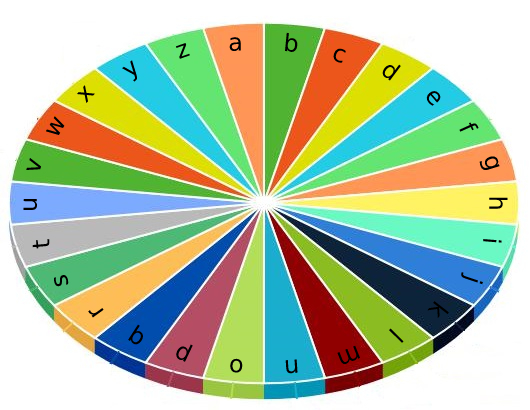1974. Minimum Time to Type Word Using Special Typewriter

#### QUESTION:

There is a special typewriter with lowercase English letters ‘a’ to ‘z’ arranged in a circle with a pointer. A character can only be typed if the pointer is pointing to that character. The pointer is initially pointing to the character ‘a’.Each second, you may perform one of the following operations:

Move the pointer one character counterclockwise or clockwise. Type the character the pointer is currently on. Given a string word, return the minimum number of seconds to type out the characters in word.

Example 1:

``````Input: word = "abc"
Output: 5
Explanation:
The characters are printed as follows:
- Type the character 'a' in 1 second since the pointer is initially on 'a'.
- Move the pointer clockwise to 'b' in 1 second.
- Type the character 'b' in 1 second.
- Move the pointer clockwise to 'c' in 1 second.
- Type the character 'c' in 1 second.
``````

Example 2:

``````Input: word = "bza"
Output: 7
Explanation:
The characters are printed as follows:
- Move the pointer clockwise to 'b' in 1 second.
- Type the character 'b' in 1 second.
- Move the pointer counterclockwise to 'z' in 2 seconds.
- Type the character 'z' in 1 second.
- Move the pointer clockwise to 'a' in 1 second.
- Type the character 'a' in 1 second.
``````

Example 3:

``````Input: word = "zjpc"
Output: 34
Explanation:
The characters are printed as follows:
- Move the pointer counterclockwise to 'z' in 1 second.
- Type the character 'z' in 1 second.
- Move the pointer clockwise to 'j' in 10 seconds.
- Type the character 'j' in 1 second.
- Move the pointer clockwise to 'p' in 6 seconds.
- Type the character 'p' in 1 second.
- Move the pointer counterclockwise to 'c' in 13 seconds.
- Type the character 'c' in 1 second.
``````

Constraints:

``````1 <= word.length <= 100
word consists of lowercase English letters.
``````

#### EXPLANATION:

easy的题目 分成三步:
1.因为每次type都是1次,所以完全可以摘出来
2.剩下就是增加一个初始a在开头
3.最后需要算出相邻两个的距离,就有两个方向, 算一下两个方向的最小值即可

#### SOLUTION:

``````class Solution {
func minTimeToType(_ word: String) -> Int {
var charArr:[Character] = ["a","b","c","d","e","f","g","h","i","j","k","l","m","n","o","p","q","r","s","t","u","v","w","x","y","z"]
var words:[Character] = Array(word)
words.insert("a", at: 0)
var result:Int = 0
for index in stride(from: 1, to: words.count, by: 1) {
var indexA:Int = charArr.firstIndex(of: words[index-1])!
var indexB:Int = charArr.firstIndex(of: words[index])!
if (indexA > indexB) {
swap(&indexA, &indexB)
}
result += min(abs(indexA-indexB), indexA + 26 - indexB)
}
return result + word.count
}
}
``````